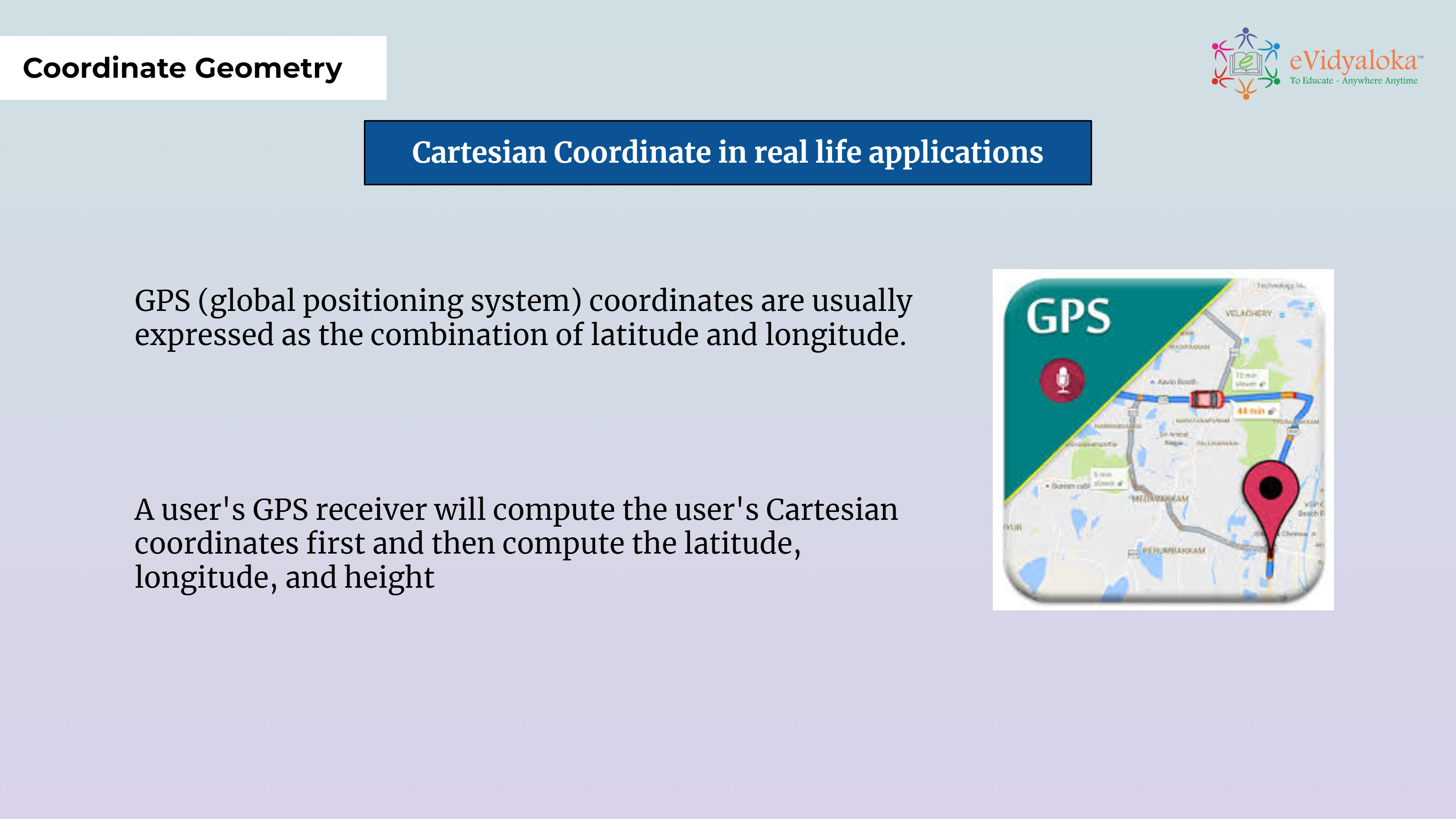# Coordinate Geometry

``````

Dear Volunteer Teacher,

Kindly teach the bridge concept of Coordinate Geometry prior to teaching the related textbook chapters.
Thank you.

## Course Content

• Introduction to the cartesian coordinate system
• Cartesian Plane
• Define Origin, x and y axis, quadrants
• cartesian coordinates (abscissa and ordinate)

## Learning Outcomes

Learning outcomes has been listed below which will serve as your guide for what students need to learn.

SWBAT:

• Understand the Cartesian Coordinate system and will know about the Rene Descartes and his contributions.
• draw the x axis and y axis and identify the quadrants
• mark the points given the coordinates and vice versa.

## PPT Deck

To make the lesson come alive, please find below PPT deck